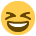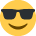# Probability Puzzle

I thought I was fairly good at maths and probabilities until I tried this GCSE one, and got stuck....

Bit embarrasing really.

A coin and a dice are thrown at the same time.

What is the probability that it will be either heads and/or more than 4.

• this one?http://www.subtangent.com/maths/flash/probsim.swf
• I would say probability is 3/12 or 25%
• I reckon:

50 % * 33.333 % = 16.7 %

or

0.5 * (2/6) = 0.166667 = 16.7 %

Or I could be completely wrong.
• Probability was never my strong point and I've no idea if this is right. but I'd work it like this

The probability of a head is 1/2 and the probability of being more than 4 is 1/3.

p(A AND= pA x pB so the probability of being a head AND over 4 is 1/2 x 1/3 = 1/6 (or 16.7%).

p(A OR= (1 - p(~A AND ~B) = 1-p(~A) x p(~B), so the probability of being a head OR over 4 is 1 - (1/2 x 2/3) = 2/3 (or 66.67%)
• oo i rememer some of how to do this

. 5 or more 2/6
. /
H 1/2 \
/
\
T 1/2

multiple 1/2 by 2/6 (i think) and the answer is 0.166 recurring

Note I've taken "more than 4" to be 5 or 6, not 4, 5 or 6.

o wait, it was and/or..... i dont have time for this, i have a maths GCSE alreadyedit it wont allow the formatting but i remember doing branch type things....
• there are thrown at the same time so they are independent

CJC is correct with the resulti love probability and stats!
• CJC wrote: »

p(A OR= (1 - p(~A AND ~B) = 1-p(~A) x p(~B), so the probability of being a head OR over 4 is 1 - (1/2 x 2/3) = 2/3 (or 66.67%)

Thats the only right answer on here!

Official answer to the GCSE question is 2/3 (or in other words .66667)

It confused me how you have to do 1 minus then the probabilities of it not being A or B. Or else in effect you end up double counting some of the outcomes. Brain baffling!

Oh and well done you annoyingly smart person!
• I couldn't remember any probability theory so I tried it using inspection as there only limited outcomes.
H1,H2,H3,H4,H5,H6
and
T1,T2,T3,T4,T5,T6

So twelve in all, of which eight satisfy the condition
8/12 = two thirds
• I couldn't remember any probability theory so I tried it using inspection as there only limited outcomes.
H1,H2,H3,H4,H5,H6
and
T1,T2,T3,T4,T5,T6

So twelve in all, of which eight satisfy the condition
8/12 = two thirds

Yes thats right also, thats the way I did it. I wanted to know the way to calculate it also.
• I started out using inspection, got the answer that way and then worked backwards to figure out the maths (then dressed it up in a bit of notation to make it look clever!).
• CJC wrote: »
I started out using inspection, got the answer that way and then worked backwards to figure out the maths (then dressed it up in a bit of notation to make it look clever!).

basically probability follows logic or if you want the operational maths signs

Logically:

P v Q = 7[(7P) ^ (7Q)]

where v = OR ^ = AND 7P means OPPOSITE of P and the rules apply as per
7(7P) = (77)P = P (two negatives = one positive)
and 7v = ^ and 7^=v

thats why the probability of an OR is the oppossite of the probability of none of the experiments happening

With operational maths signs:

a - b = - [ (-a) + (-b)] where - = OR and += AND